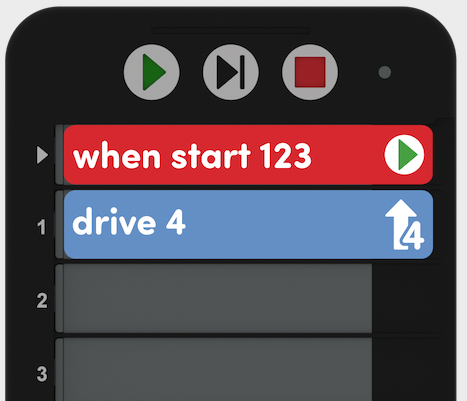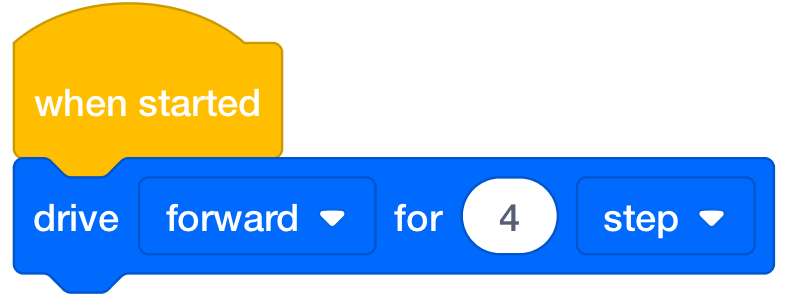Skip to main contentNumber Line
Unit Alternate Coding Methods

Teacher Portal

# Alternate Coding Methods

## Adapt this Unit to Use an Alternate Coding Method

This STEM Lab Unit is written to use the buttons on the 123 Robot to touch to code. However, it can also be completed using the Coder or VEXcode 123. This flexibility can help you meet the individual needs of your students. For example, if your students are familiar with VEXcode 123, they can complete all the coding activities with VEXcode 123 to move the robot on the number to solve an equation. The Summary section of each Lab contains additional information that can help you modify the individual Lab to use VEXcode 123.

### Coder Adaptation

If using the Coder with this Unit, give each group a Coder and a set of Motion cards. The following Motion cards are recommended:123 Robot will drive forward 1 robot length (1 step).123 Robot will drive forward 2 robot lengths (2 steps).123 Robot will drive forward 4 robot lengths (4 steps).

For more information about how to use the Coder, see the Using the VEX Coder Knowledge Base article.

In both Labs, students will drive the 123 Robot on a number line to solve an equation. Coder project solutions will depend on the equations that students are given. Here is a possible Coder solution for the equation: 2+4=6.  Have students place the 123 Robot on the number 2 on the number line, and make sure the front arrow of the robot is pointing to the higher numbers. Then, press the Start button to begin the project.Possible Solution for equation  2 + 4 = 6

### VEXcode 123 Adaptation

You can also adapt this Unit to use VEXcode 123.  Students can use the [Drive for] block for the coding activities in this Unit. The [Drive for] block moves the 123 Robot forward or in reverse a given distance. Set how far the 123 Robot will move by entering a value into the oval. For more information on how to work with VEXcode 123, see the VEXcode 123 Section of the Knowledge Base.[Drive for] block

Here is a possible VEXcode 123 solution for the equation: 2+4=6. Have students place the 123 Robot on the number 2 on the number line, and make sure the front arrow of the robot is pointing to the higher numbers. Then, press the Start in the Toolbar to begin the project.Possible VEXcode Solution for Equation 2 + 4 = 6